# Collisions, Impulses and Impulsive Tension

• SilverSoldier
In summary, when an object attached to a fixed point with a string is given a velocity and the string goes taut, the component of the velocity of the particle becomes zero in the direction along the string. This is because the tension in the string causes the particle to come to a halt in that direction. The same principle applies when a velocity is given to one of two objects connected with a string, and when impulsive tensions act in the presence of other forces. In the case of a collision between two objects with external forces, the particles will move with equal and opposite velocities after the collision, unless there is friction involved. The tension in the string can be calculated using the initial and final velocities of the particles and the impulse experienced. Itf

#### SilverSoldier

Homework Statement
There are several concepts regarding these topics topic that I do not quite grasp well, that I have detailed below.
Relevant Equations
Impulse = Change in momentum (##I = \Delta mv##)
Law of Conservation of Momentum
Newton's Law of Restitution
Conservation of Energy
1. When an object attached to a fixed point with a string, is given a velocity and the string goes taut.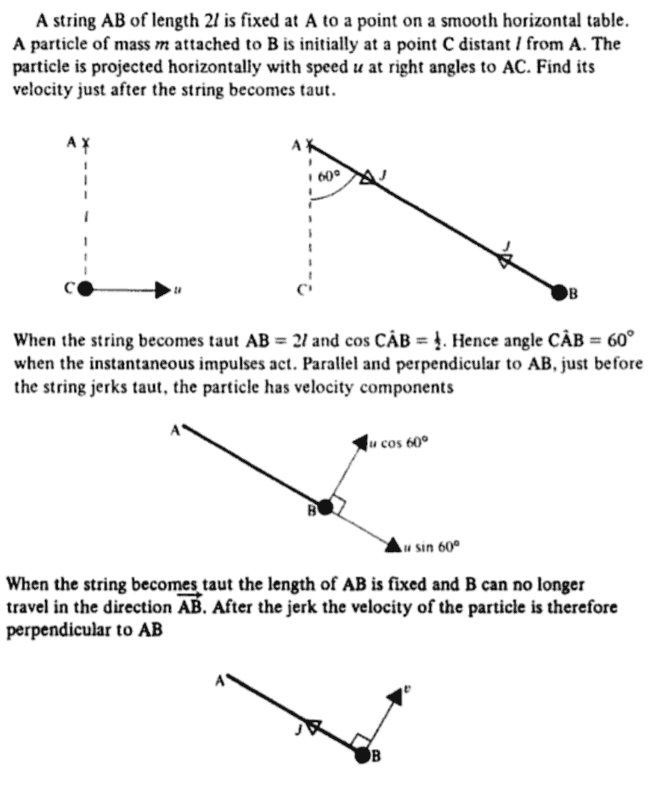So it says in this book (Applied Mathematics 1 by L. Bostock and S. Chandler) that when the string goes taut, the component of the velocity of the particle becomes zero in the direction along the string.
1. Why must that be the case? Is it not possible that the particle acquires a velocity in the opposite direction, i.e., along ##BA##, according to the example given?​
2. If the velocity does for some reason become zero, what will happen immediately after the particle acquires this velocity? Is it only for as long as the impulse acts that there will be a tension in the string? Will there also be a tension after the impulse? How can it be calculated? Will the particle move in a circular path, and the tension be ##\dfrac{mv^2}{2l}##?​
2. When a velocity is given to one of two objects are connected with a string, and the string goes taut.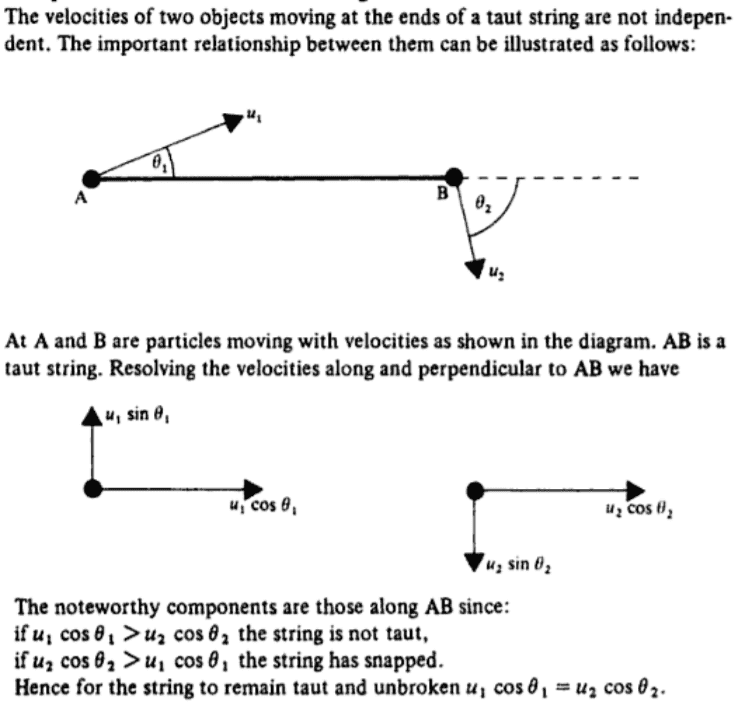The same book states that the final velocities along the direction of the string will be the same after an impulsive tension is experienced. Again, why must this be the case? What is wrong with the string not being taut? Can ##A## not move faster than ##B## after the impulse, and why?

3. When impulsive tensions act in the presence of other forces.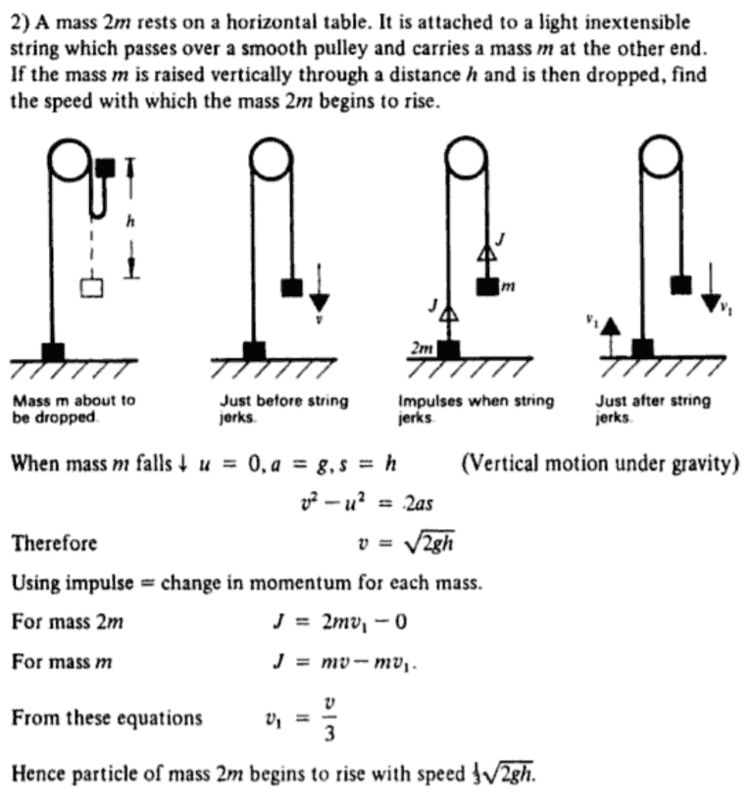1. In this example, don't the weights of the particles have any effect on the impulse that arises?​
2. Why do both particles acquire the same velocity due to the impulse?​
3. What happens after these particles acquire these velocities? Will there be a tension present in the string? Is it possible to calculate it? Will both particles accelerate as a system with the same accelerations, or will they accelerate differently?​
4. In a situation such as the following, where, say ##A## is thrown down with some velocity ##u## while ##B## is dropped from rest, and the string goes taut, what will happen? Can the above equations still be used? Does the presence an impulse and an external force along different directions, unlike in the pulley example above affect the problem in any way?​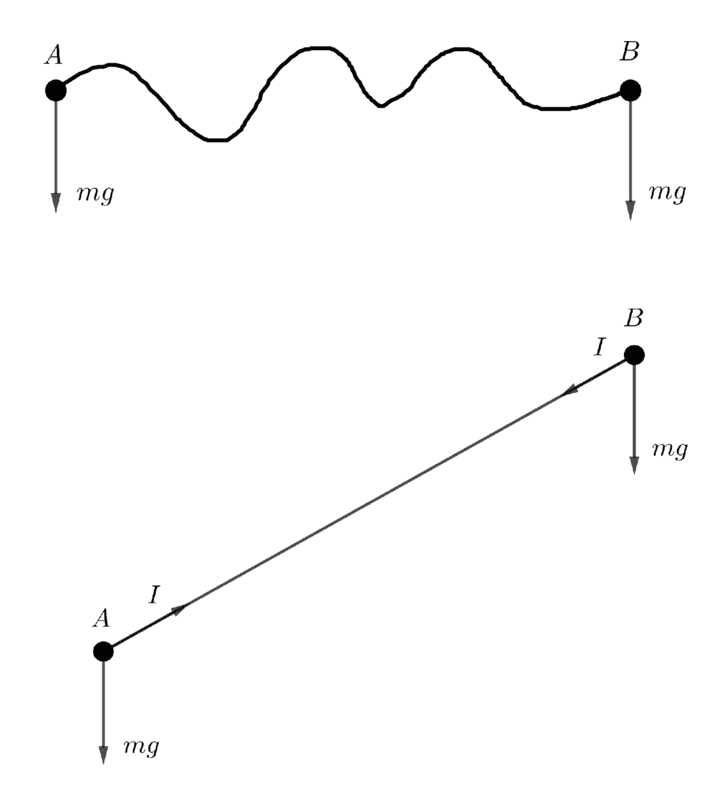4. When two objects with external forces acting on them collide.
1. If a particle ##P## that has a constant force acting on it, collides with another particle ##Q## moving at constant velocity, how will the particles move? Will they accelerate as a system?
2. If ##P## is released from the top of an incline, and ##Q## is projected up along the incline at some velocity ##u##, what can we do to find out the velocities of the particles after the collision? If ##P## were instead thrown down the incline, it possible that after collision ##P## acquires a velocity greater than what ##Q## acquires, in the same direction? How will the particles move in such a situation?​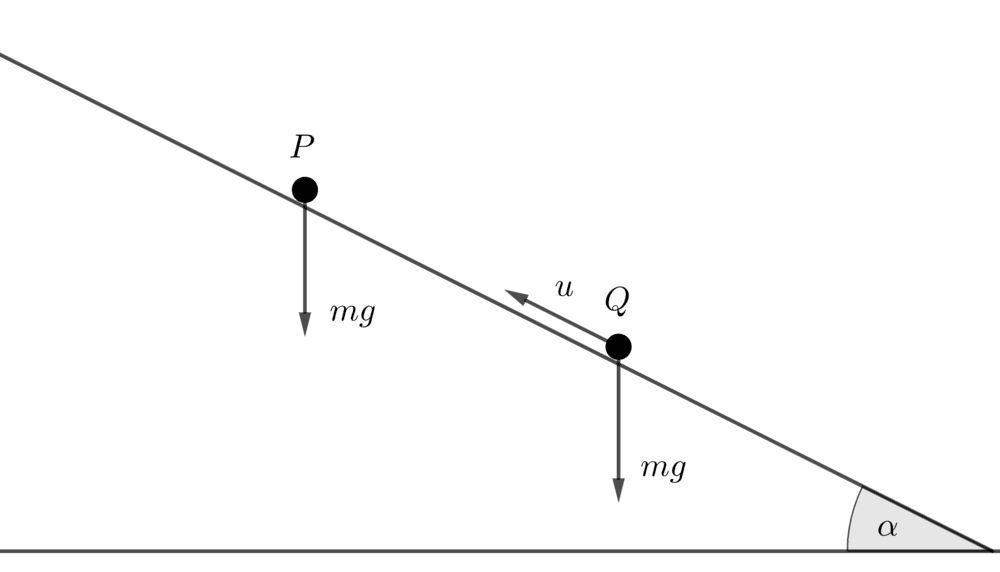•Delta2
Why must that be the case? Is it not possible that the particle acquires a velocity in the opposite direction, i.e., along BA, according to the example given?
The question omitted to specify inelastic.
what will happen immediately after the particle acquires this velocity? Is it only for as long as the impulse acts that there will be a tension in the string? Will there also be a tension after the impulse? How can it be calculated? Will the particle move in a circular path, and the tension be mv22l?
You can calculate this easily enough. The impulse no longer matters; you just have the two masses moving with equal and opposite velocities, so the tension and accelerations will be the same as for being released from rest.
don't the weights of the particles have any effect on the impulse that arises?
If the impact is taken as lasting negligible time then the impulse due to other forces, like gravity, is also negligible: p=∫F.dt.
For the duration of the impact, the impact forces are much larger.

You do have to be careful if there is friction. If a ball is thrown horizontally, not spinning, and lands on a rough surface, then the vertical impulse generates a corresponding frictional impulse.
Why do both particles acquire the same velocity due to the impulse?
Again, the question should specify inelastic. "Inextensible" doesn't cover it.
What happens after these particles acquire these velocities?
As before, easily calculted.
Does the presence an impulse and an external force along different directions, unlike in the pulley example above affect the problem in any way?
Again, negligible impulse during the impact.

••jbriggs444 and SilverSoldier
You can calculate this easily enough.

Mmm, do you mind telling me how?How can the tension that arises in the string be calculated, knowing only their initial and final velocities, and the impulse experience? By the way, in case it was not clear, I am not asking about the tension that arises in the string while the particle experiences the impulse, but what happens to the particle after the impulse finishes acting on the string.

The impulse no longer matters; you just have the two masses moving with equal and opposite velocities

Also, point ##A## is fixed, and ##A## is not a movable mass, as I think you've mistakenly assumed.

We only know that the particle will have a velocity perpendicular to the string by the time that the impulse finishes acting, and that the string, I assume, will then be just taut.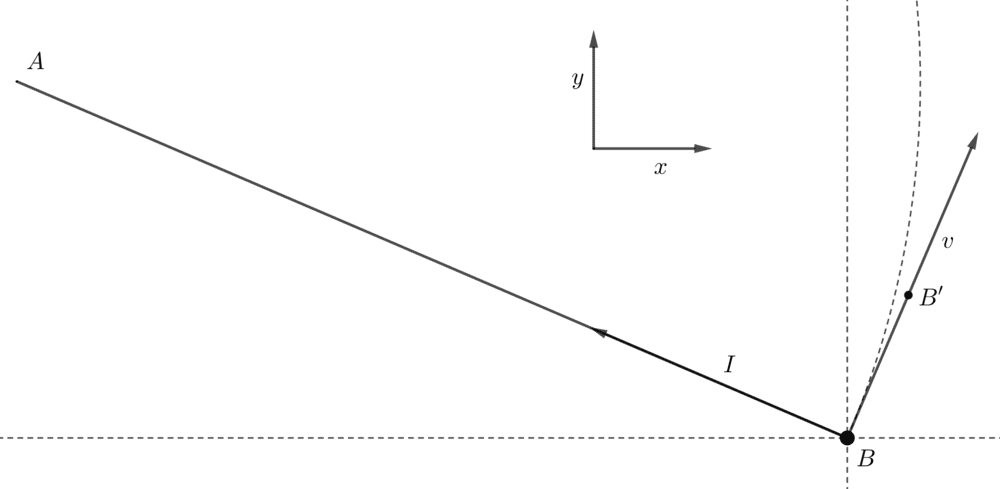If the particle should move with the uniform velocity it acquires, it must come to some such point as ##B'##, as indicated above, but it can't because the string is not that long. Will there also act another impulse along the ##x## direction, so the particle shall only move in the ##y## direction? Or will the particle start to move in a circular path?

Again, the question should specify inelastic. "Inextensible" doesn't cover it.

But aren't "inelastic" and "inextensible" the same thing? How are they different? If the question did specify "inelastic", how would the solution be different?

•Delta2
Mmm, do you mind telling me how?How can the tension that arises in the string be calculated, knowing only their initial and final velocities, and the impulse experience? By the way, in case it was not clear, I am not asking about the tension that arises in the string while the particle experiences the impulse, but what happens to the particle after the impulse finishes acting on the string.
As @haruspex wrote, you know how the objects are constrained to move after the collision. You know their velocities immediately following the impulsive event. So you can calculate how much tension is required to have them continue to move in the required manner.

•SilverSoldier
As @haruspex wrote, you know how the objects are constrained to move after the collision. You know their velocities immediately following the impulsive event. So you can calculate how much tension is required to have them continue to move in the required manner.

What do you mean by tension required to have them move in the required manner? We do not get to decide how they move after an impulse, do we? How will they move?

From what I understand, they can either move in the ##y## direction, or move in a circular path. How do we know which of these happens? And if it should move in a circular path, how do we know whether or not it will do so with uniform angular velocity?

What do you mean by tension required to have them move in the required manner? We do not get to decide how they move after an impulse, do we? How will they move?

From what I understand, they can either move in the ##y## direction, or move in a circular path. How do we know which of these happens? And if it should move in a circular path, how do we know whether or not it will do so with uniform angular velocity?
That is why @haruspex mentioned that the problem should specify an inelastic collision. Then you know that the radial velocity is reset to zero by the impulsive event. We know that there are no tangential forces. So we know that the angular velocity (or, at least, the angular momentum) will be uniform.

Given a non-zero initial tangential velocity and a zero initial radial velocity, the path taken by an object on a rope as it continues in motion is pretty clear.

In the real world, there are differences in ropes. A hemp rope will give you a pretty inelastic collision. With nylon, you'll get a bounce.

Last edited:
•SilverSoldier and Delta2
@jbriggs444 @haruspex i want to ask the same thing as at the end of post #3, why inelastic isn't the same thing as inextensible?

@jbriggs444 @haruspex i want to ask the same thing as at the end of post #3, why inelastic isn't the same thing as inextensible?
A perfectly inextensible rope is one that can deliver an infinite(*) force with a zero displacement. If you try to calculate the energy absorbed by such a rope, you will wind up multiplying zero and infinity. The result is not defined.

It could be that no energy is absorbed and the object bounces back. It could be that all of the energy is absorbed and the object stops dead. The fact that the rope is inextensible does not help us decide which it is.

Or consider that inextensible ropes are idealizations that do not really exist. So take the limit of a rope that gets stiffer and stiffer. No matter how stiff the rope gets, it can still be either perfectly elastic, perfectly inelastic or something in between. So you need to track elasticity separately from stiffness.

Elasticity, one might express as a coefficient of restitution. Stiffness, one might use the k from Hooke's law.

In practice, for objects that are very stiff, elasticity tends to become very high. e.g. a hard steel ball bearing on a thick steel plate has a coefficient of restitution nearly equal to 1.

As I recall, one can trace this to a high speed of sound. So the collision deflects a large local volume of material at a low relative velocity. This means that the vibrational energy in the impacting objects' material is low. Not much energy is absorbed, so the bulk kinetic energy of the participants is largely conserved.

(*) If the inextensible rope had a finite breaking strength then it could not absorb any energy whatsoever and we would be left asking: "where did the energy go?". We would be no closer to predicting whether or not a rebound would ensue.

Last edited:
•SilverSoldier
Thank you very much for your responses. I guess most of my doubts are now clarified.It could be that no energy is absorbed and the object bounces back. It could be that all of the energy is absorbed and the object stops dead. The fact that the rope is inextensible does not help us decide which it is.

So in the event that the two particles are connected with a perfectly elastic string, we can use the Law of Conservation of Momentum along and perpendicular to the direction of the string, and Newton's Law of Restitution to obtain three equations (considering the ##x## and ##y## components of one particle's velocity separately), and solve for the final velocities of the two particles, is that right?

I suppose the string goes slack immediately after providing the impulse because one particle moves in the direction of the string?

We know that there are no tangential forces. So we know that the angular velocity (or, at least, the angular momentum) will be uniform.

Given a non-zero initial tangential velocity and a zero initial radial velocity, the path taken by an object on a rope as it continues in motion is pretty clear.

Oh yes, there are no tangential forces. It just didn't occur to me that that implies uniform circular motionAlso, in a case like the pulley problem in (3), will the masses both accelerate as a system, so that they decelerate initially, come to rest, and then accelerate, without the string jerking a separate time? If the greater mass comes to rest after hitting the ground, its acceleration will change suddenly. That is not an impulse, is it?

there are no tangential forces. It just didn't occur to me that that implies uniform circular motion
For uniform circular motion you also need that the normal force is constant.
in (3), will the masses both accelerate as a system, so that they decelerate initially, come to rest, and then accelerate, without the string jerking a separate time?
Yes.
If the greater mass comes to rest after hitting the ground, its acceleration will change suddenly. That is not an impulse, is it?
Its velocity will also change suddenly, so yes it is an impulse on that mass, but not on the other mass (which will be in free fall until it returns to the same height, whereupon, etc.).

•SilverSoldier
For uniform circular motion you also need that the normal force is constant.
When the movement of these particles is on a smooth surface, the normal force is anyway constant, right?

t could be that no energy is absorbed and the object bounces back. It could be that all of the energy is absorbed and the object stops dead. The fact that the rope is inextensible does not help us decide which it is.
Well when i have in my mind an inextensible string i automatically think of the second case (all energy absorbed). But ultimately probably you are right, inextensibility doesn't imply this second case.

When the movement of these particles is on a smooth surface, the normal force is anyway constant, right?
Depends on the shape of the surface.

Well when i have in my mind an inextensible string i automatically think of the second case (all energy absorbed). But ultimately probably you are right, inextensibility doesn't imply this second case.
As @jbriggs444 noted, idealisations should be thought of as limiting cases of more realistic versions. If the behaviour in the real world converges in that limit then we can take that as the behaviour of the idealisation.
Real strings have some extensibility and elasticity. We can model this as two different spring constants, ##k_{stretch}\geq k_{relax}##.
To model an inelastic string, set ##k_{relax}=0##. If it is also inextensible then let ##k_{stretch}\rightarrow\infty##.
To model an inextensible but perfectly elastic string, set ##k_{stretch}=k_{relax}##, then let ##k_{stretch}\rightarrow\infty##.

Correction: I should have written let ##k_{stretch}\rightarrow k_{relax}##, not set ##k_{stretch}=k_{relax}##

Last edited:
To model an inextensible but perfectly elastic string, set kstretch=krelax, then let kstretch→∞.
I can't understand how a string can be inextensible but perfectly elastic but ok i don't want here to intervene with my understanding problems, i think the OP has enough :D.

I can't understand how a string can be inextensible but perfectly elastic but ok i don't want here to intervene with my understanding problems, i think the OP has enough :D.
No actual string can be either truly inextensible or perfectly elastic. As I wrote, (or should have written) it is the limit as ##k_{relax}\rightarrow k_{stretch}## and both tend to infinity. For this to be valid, it is only necessary that, in principle, ##k_{relax}## can reach very high values.

•Delta2
@haruspex I don't understand how you define ##k_{relax}## in first place. When we try to stretch a string then we might say that it behaves as some sort of spring with ##k_{stretch}## but when we move to the opposite direction I don't think that the string behaves like a spring, it mostly behaves as it doesn't exist.

@haruspex I don't understand how you define ##k_{relax}## in first place. When we try to stretch a string then we might say that it behaves as some sort of spring with ##k_{stretch}## but when we move to the opposite direction I don't think that the string behaves like a spring, it mostly behaves as it doesn't exist.
It's krelax, not kcompress.

•Delta2